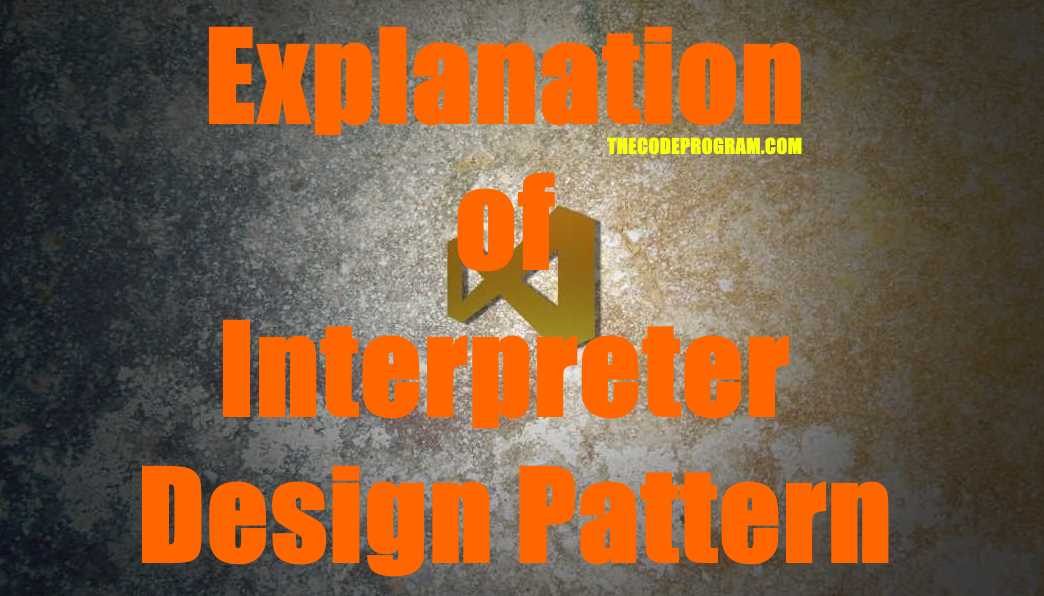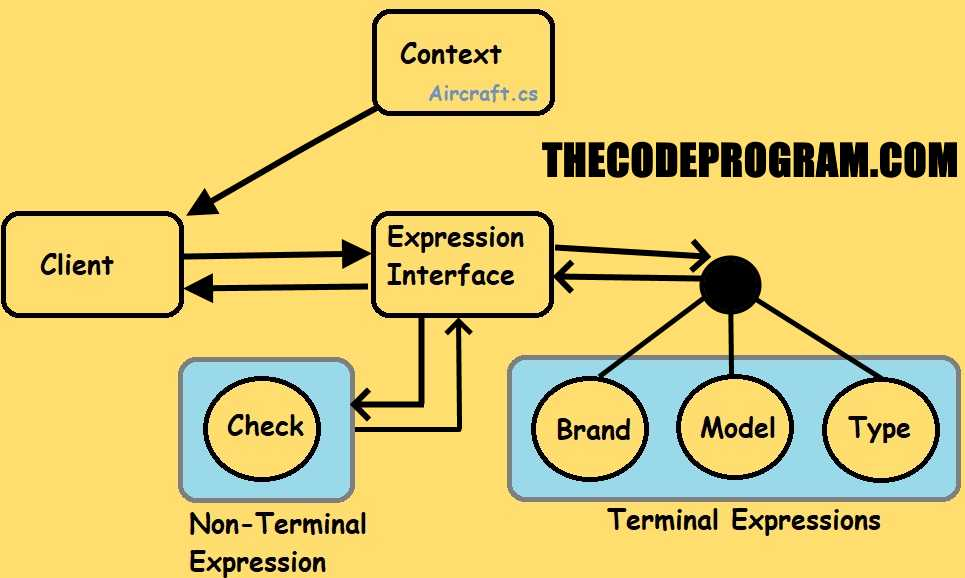## Explanation of Interpreter Design Pattern

Hello everyone, in this article we are going to talk about Interpreter Design Pattern within Behavioral Design Patterns. We are going to explain it and we will make an example in C#.

Let's get started.

Firstly, I want to talk about What is Interpreter Design Pattern?

Interpreter Design Pattern can be used when we need to process a string that have a language pattern according to logical or numerical structures.

We need to see some of terms before starting. These terms are explains the purpose and logic of Interpreter design pattern.

• Client : This is program that send the context to the Expression operator and get the results.
• Context: The data which will be interpreted.
• AbstractExpression : It provides the rules of interpreting. It referances the expressions for the interpreting.
• TerminalExpression : ıt referances the interpret operations withing the given string.with characters inside the string. They have the exact meanings.
• NonterminalExpression : Non-terminals never actually appear in the linear input sequence, only exist in grammar rules

Below image you can see the Schematic of the Interpreter Design Pattern with my example:I want to talk about differances between Terminal and NonTerminal Expressions a little bit with examples.

The Non-Terminal expressions are refers the rules which you can not see them in the string but we know they are exist. For example let's say a greetings sentence like Hello, how are you? In here Hello, you is terminal expressions. Because you can see them in the string.

But in this sentences you can not see the word greeting and sentence. So here the greeting and sentence are non terminal expression while hello and you are terminal expression.

Now Let's make our example application.

In this example I am going to make this program to interpret the aircraft brand, type and model number from its service model name.

First I will create a context class. In this context class I will keep the aircraft model names and some required methods to decide what it is.

Context.cs

``````
namespace InterpreterPattern_Example
{
class Context
{
private string ac_model = "";
private bool isAircraft = false;

public Context(string _ac_model)
{
this.ac_model = _ac_model;
}

public string getModel()
{
return this.ac_model;
}

public int getLenght()
{
return this.ac_model.Length;
}

public string getLastChar()
{
return this.ac_model[this.ac_model.Length - 1].ToString();
}

public string getFirstChar()
{
return this.ac_model.ToString();
}

public void setIsAircraft(bool _isAircraft)
{
this.isAircraft = _isAircraft;
}

public bool getIsAircraft()
{
return this.isAircraft;
}
}
}
``````

After this I created an Expression interface to derive all Expression Classes.

Expression.cs

``````
namespace InterpreterPattern_Example
{
interface Expression
{
void InterpretContext(Context context);
}
}
``````
And below My Expression Classes which derived from above interface.
We assume the aircraft models only start with A or B and contains 4 or 5 chars.

CheckExpression

``````
using System;
//In this class we are going to check if the entered text is an aircraft
namespace InterpreterPattern_Example
{
class CheckExpression : Expression
{
public void InterpretContext(Context context)
{
//We assume tthe aircraft models only start with A or B and contains 4 or 5 chars.
string ac_model = context.getModel();
if (ac_model.StartsWith("A") || ac_model.StartsWith("B"))
{
if (ac_model.Length == 4 || ac_model.Length == 5)
{
context.setIsAircraft(true);
Console.WriteLine(ac_model + " is an aircraft...");
}
else
{
context.setIsAircraft(false);
Console.WriteLine(ac_model + " is not aircraft...");
}
}
else
{
context.setIsAircraft(false);
Console.WriteLine(ac_model + " is not aircraft...");
}
}
}
}
``````

To check entered aircraft model we assume only Airbus or Boeing. So it can be started with only A and B.

BrandExpression.cs

``````
using System;

namespace InterpreterPattern_Example
{
class BrandExpression : Expression
{
public void InterpretContext(Context context)
{
if (context.getIsAircraft() == true)
{
if (context.getFirstChar().Equals("A"))
Console.WriteLine("Brand is Airbus");
else if (context.getFirstChar().Equals("B"))
Console.WriteLine("Brand is Boeing");
}
else
Console.WriteLine("Brand could not be interpreted");
}
}
}
``````

First character of the entered model will refer to brand and later 3 numbers are refer to the brand model of the aircraft.

ModelExpression.cs

``````
using System;

namespace InterpreterPattern_Example
{
class ModelExpression : Expression
{
public void InterpretContext(Context context)
{
if (context.getIsAircraft() == true)
{
Console.WriteLine("Model is : " + context.getModel().Substring(1, 3));
}
else
Console.WriteLine("Model could not be interpreted");
}
}
}
``````

If the aircraft brand model ending with "F", it refers to Freighter aircraft so for cargo operations.

TypeExpression.cs

``````
using System;

namespace InterpreterPattern_Example
{
class TypeExpression : Expression
{
public void InterpretContext(Context context)
{
if (context.getIsAircraft() == true)
{
string ac_model = context.getModel();
if (context.getLenght() == 5 && context.getLastChar().Equals("F"))//F-> Freighter
{
Console.WriteLine("Aircraft type is Cargo/Freighter");
}
else
Console.WriteLine("Aircraft type is Passenger Transportation");
}
else
Console.WriteLine("Type could not be interpreted");
}
}
}
``````

As you can see above our Expression classes are ready. With above classes we check if it is an aircraft and if it it correct we interpreted its brand model and type with related terminal characters.

Now we have to call these methods from Program.

Program.cs

``````
using System;
using System.Collections.Generic;

namespace InterpreterPattern_Example
{
class Program
{
static void Main(string[] args)
{
Console.Title = "Interpreter Design Pattern Example - TheCodeprogram";

List<Context> lstAircrafts = new List<Context>();
List<Expression> lstExpressions = new List<Expression>();

for (int ac_index = 0; ac_index < lstAircrafts.Count; ac_index++)
{
for (int exp_index = 0; exp_index < lstExpressions.Count; exp_index++)
{
lstExpressions[exp_index].InterpretContext(lstAircrafts[ac_index]);
}
Console.WriteLine("-----------------------------------");
}
}
}
}
``````
As you can see above I have created two Collection Lists. One of the will keep my contexts the other one will hold the expression classes. I wrote two for loops and inside of them I will I will interpreted the aircraft models with expressions in order, one by one.

You can reach the example application on Github : https://github.com/thecodeprogram/InterpreterPattern_Example

I wish you all healthy days.

Have a good interpreting the texts.

Burak Hamdi TUFAN

02/04/2020

05/04/2020

16/07/2020